### Fourier Series

Chapter 3 Complex Foourier Series
Chapter 3.1 Complex Foourier Series exp(jωt) as a rotating vector
You always meet this function in electricity, automatics, acoustics…
See animation. You return to article by typical windows pushbutton “previous site return”Fig. 3-1 Complex function z=1exp(jωt) as roatating vector ω=2.62/sec
Click  ANIMATION–>author Chetvorno
You see rotating black dot. Check the time of the 10 rotations, t=24sec–>T=2.4sec –>pulsation ω=2π/T=2.62/sec
The z=1exp(jωt) black dot location is just a complex function  in the complex numbers plane.
For example:
1exp(j0)=1+j0=1
1exp(j60º)=1exp(jπ/3)≈0.5+1+j0.8
as Fig.3-1 (assume that ωt=π/3=60º)
You can use Euler formula 1exp(jωt)=sin(ωt)+jcos(ωt) too:
For example:
1exp(j0)=1+j0=1
1exp(jπ/2)≈0+j1=1j

Chapter 3.2 Complex Foourier Series c*exp(jωt) as a rotating vector
The previous coeffient c was a real number c=1. Let’s consider any complex number, i.e. c=1.8+0.4j for example.Fig. 3-2
c*exp(jωt)
as rotating vector
The real number 1 is rotating as a exp(jωt).
The complex  number c=1.8+0.4j is rotating as a c*exp(jωt).

Chapter 3.3 a1cos(1ωt) i b1sin(1ωt) components as rotating vectors.
Let’s consider components a1cos(1ωt) and b1sin(1ωt)
It enables easy Tigonometric Fourier Series to Complex Fourier Series transformation.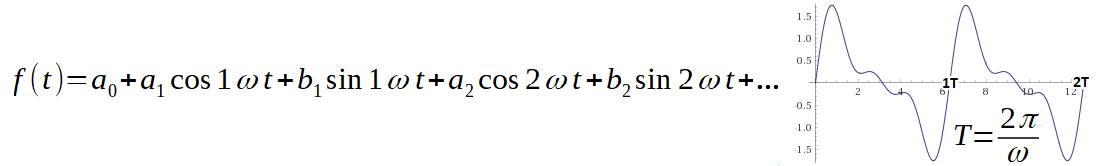Fig.3-3
a0-constant
a1,b1,a2,b2…-appropriate cosinus/sinus amplitudes
Are the a0,a1,b1,a2,b2…any value?—> The Fig.3-3 is a  common formula for cosinus/sinus sum with different a1,b1,a2,b2…amplitudes and 1ω, 2ω, 3ω… pulsations.
Are the a0,a1,b1,a2,b2… calculated acc. Fig. 2-12 Chapter 2.2 formula?—>The Fig.3-3 is a Tigonometric Fourier Series formula.
Let’s consider a1=1, b1=0.7 and ω=2.62/sec.
I.e. We are looking rotating vectors for:
f(t)=1cos(2.62t)+0.7sin(2.62t)
The vectors may be treated as complex numbers here.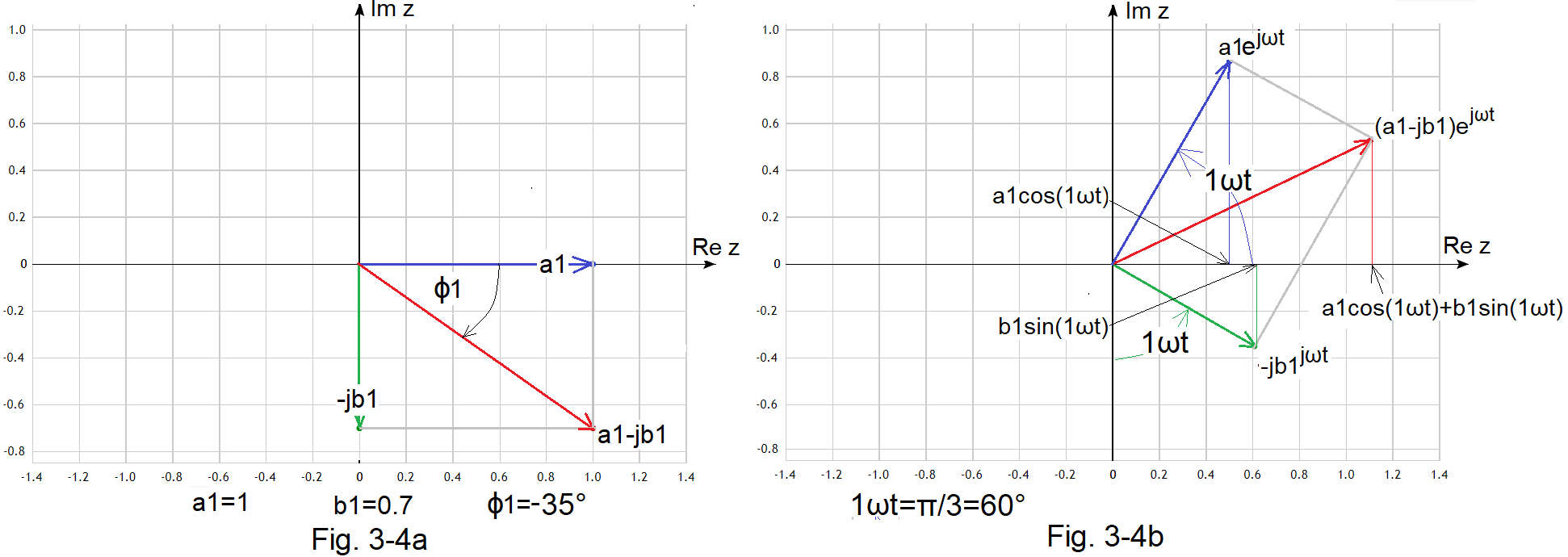Fig.3-4
a1cos(1ωt) and b1sin(1ωt) components as vertical projection for real axis Re z
Fig. 3-4a
Rotating vectors z1z2 and the sum c1 intial state i.e. for 1ωt=0.
z1=a1=1
z2=-jb1=-j0.7
c1=z1+z2=1-j0.7
–>see*Note
Fig. 3-4
b
Rotating vectors z1z2 and the sum c1 after time t when 1ωt=π/3=60º
z1*exp(j1ωt)=a1*exp(jωt)=1*exp(j1ωt)
z2*exp(jωt)=-jb1*exp(1jωt)=-j0.7*exp(j1ωt)
c1=z1+z2=(a1-jb1)*exp(j1ωt)=(1-j0.7)*exp(j1ωt)
The crucial conclusion
The a1cos(1ωt)+b1sin(1ωt) is a rotating vector (a1-jb1)*exp(exp(j1ωt) vertical projection for real axisRe z
Or other words
a1cos(1ωt)+b1sin(1ωt) is a real part of the complex function (a1-jb1)*exp(exp(j1ωt)
Or other words
a1cos(1ωt)+b1sin(1ωt) =Re z{ (a1-jb1)*exp(j1ωt)}
The evidence is the Fig. 3-4b school trigonometry where
blue and green vectors lengths are:
a1=1 and b1=0.7.
These rotating vectors represent the f(t) functionFig. 3-5
The f(t)=1.221cos(2.62t-35º)=1cos(2.62t)+0.7sin(2.62t) as rotating vectors from Fig-3-4       ω=2.62/sec
*Note
c1=1-j0.7
may be presented as module |c1|=1.221 and phase φ1≈-35º or equivalently as 1.221*exp(-j35º).
Module 1.221 is a 1 and 0.7 Pitagoras and φ1=arctg(-0.7/1)≈-35º. Use calculator to check it.

Chapter 3.4 Complex Fourier Series for 3 harmonics
Chapter 3.4.1 Version with vertical projection for real axis Re z
We start with f(t) function with harmonics only. This function is easier to analyse.
f(t)=a1cos(1ωt)+b1sin(1ωt)+a2cos(2ωt)+b2sin(2ωt)+a3cos(3ωt)+b3sin(3ωt)
Some questions are coming up now.
First
Why do fragmentize the f(t) for Fourier harmonics? This function consists of  Fourier harmonics, after all! You see a0, a1,b1,a2,b2 a3,b3 coefficients in f(t) and you don’t need to use the previous chapter Fig. 2-12 formula. Yes, you are right. But the reason is didactical only.
In any case, the formula may be used and the result is the same!
Second
Why “Fourier Series for 3 harmonics”. We see components in f(t). Because a1cos(1ωt)+b1sin(1ωt) may be treated as 1 harmonic c1*sin(1ωt-φ1) for example.
Let’s f(t) is a particular function:
f(t)=1cos(1ωt)+0.2sin(1ωt)+0.6cos(2ωt)+0.4sin(2ωt)+0.4cos(3ωt)+0.4sin(3ωt)
i.e.
a1=1 b1=0.2
a2=0.6 b2=0.4
a3=0.4 b3=0.4
The basic pulsation ω may be any. But if you like nitty-gritty–>ω=2.62/sec as in animation Fig. 3-1.

c1, c2, c3
are vectors or complex numbers:
c1=a1-jb1=1-0.2j
c2=a2-jb2=0.6-0.4j
c3=a3-jb3=0.4-0.4j
The f(t) function may be assign the rotating vectors or complex numbers now.
c1*exp(j1ωt)
c2*exp(j2ωt)
c3*exp(j3ωt)
The conclusion come from Fig. 3-4 now
a1cos(1ωt)+b1sin(1ωt)=Re z {(a1-jb1)*exp(j1ωt)} >i.e. real part of (a1-jb1)*exp(j1ωt)
Analogously for 2ωt,3ωt
a2cos(2ωt)+b2sin(2ωt)=Re z {(a2-jb2)*exp(j2ωt)}
a3cos(3ωt)+b3sin(3ωt)=Re z {(a3-jb3)*exp(j3ωt)}
I.e.
f(t) time function is a real part of the rotating vectors sum
c1*exp(j1ωt)+c2*expj2ωt)+c3*exp(j3ωt)
I.e.
a1cos(1ωt)+b1sin(1ωt)+a2cos(2ωt)+b2sin(2ωt)+a3cos(3ωt)+b3sin(3ωt)=Re z {c1*exp(j1ωt)+c2*exp(j2ωt)+c3*exp(j3ωt)}
when c1=a1-jb1, c2=a2-jb2, c3=a3-jb3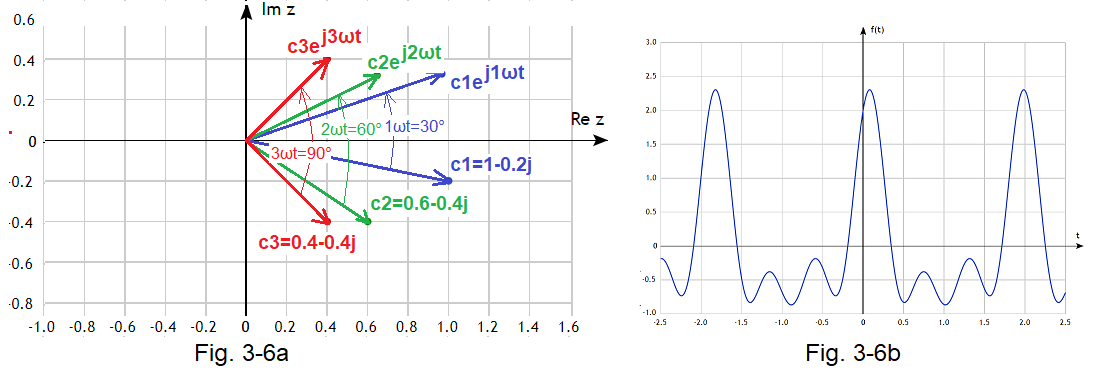Fig. 3-6
Fig. 3-6a Rotating vectors of the f(t) function
Fig. 3-6b f(t) function as a harmonics sum
c1, c2, c3  rotating vectors initial state when t=0
c1*exp(j1ωt), c2*exp(j2ωt), c3*exp(j3ωt) rotating vectors when 1ωt=30º (or 2ωt=60º or 3ωt=90º)
The “most delayed” vector c3 did the biggest rotation after time t (when 3ωt=90º). No wonder, it has the biggest pulsation 3ωt!
The rotating vectors sum vertical projection  for Re z is just a f(t) time function!
I.e.
f(t)=Re z  {c1*exp(j1ωt)+c2*exp(j2ωt)+c3*exp(j3ωt)}
Two private notes:
1.
The rotating vectors returned to its initial state after T=2π/ω. But blue did 1 rotation, green 2 rotations and red rotations.
2. The vectors are similar to Solar system with Sun in center (0,0) and orbits:
c3*exp(j3ωt)-
Venus orbit (the least)
c2*exp(j2ωt)Earth
orbit
c1*exp(j1ωt)-Mars
orbit  (the biggest)
Fig. 3-6 shows rotating vectors but you don’t see their sum!
Fig. 3-7 is without this fault. The rotating vectors sum is the end of the c3*exp(j3ωt).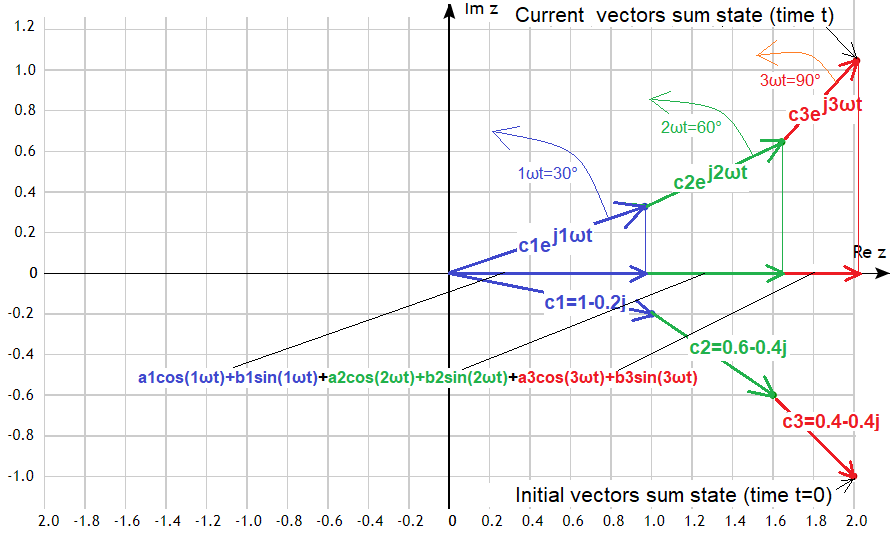Fig. 3-7
f(t)=1*exp(j1ωt) + c2*exp(j2ωt) + c3*exp(j3ωt) as the end of red rotating vector
-The blue rotates around  (0,0)
-The green rotates around the  blue end
-The red rotates around the green end
It’s similar to the cracking whip when its end exceeds sound barrier.
Blue is an Earth rotating around Sun
Green is a Moon rotating around Earth
Red
is a satellite rotating around Moon
Go for a moment to YouTube Fig. 3-16. All will be clear.
Conclusions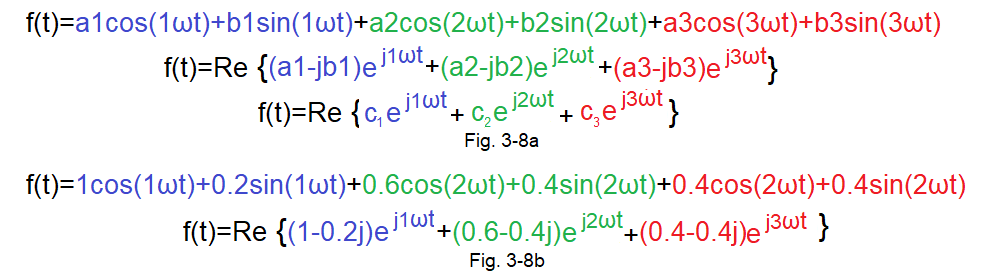Fig. 3-8
Complex Fourier Series formula for f(t) with harmonics. Version with vertical projection-another words “With Re z” formula
Fig. 3-8a
General formula when any harmonics
Fig. 3-8b
Particular formula when 3 concrete harmonics

Chapter 3.4.2 Oppositely rotating vectors version. Other words -“without Re z” version
This is most popular Complex Fourier Series version. So, it is often and simly named Complex Fourier Series formula.Fig. 3-9
Fig.3-9a
and Fig.3-9b formulas are equivalent!
When we make changes in the formula Fig. 3-9a
1. Rotating (1-0.2j)*exp(j1ωt) vector will be replaced by the sum of
– it’s half –>(0.5-0.1j)*exp(j1ωt)
– it’s conjugate half–>(0.5+0.1j)*exp(-j1ωt)
2. We do analogously with (0.6-0.4j)exp(j2ωt) and (0.4-0.4j)exp(j3ωt)
then
the Fig. 3-9b formula is equivalent.
Note, that Fig. 3-9b formula is double bigger, but the result is immadietaly real, without Re{…} operation!
Fig. 3-9c is a general formula. Remember that c1, c2 and c3 are the halfs of the Fig. 3-8a c1, c2 and c3 coefficients!Fig. 3-10
f(t) as oppositely rotating vectors sum
A – general formula for any c(-3)c(-2), c(-1)c1, c2 c3
– particular formula for concret c(-3)c(-2), c(-1)c1, c2 c3
You see that:
1. Lower rotatting vectors are halfs of the Fig.3-7 lower rotatting vectors  for t=0
2. Lower and higher vectors are conjugate complex numbers
3. Lower and higher vectors are contrary rotating and their sum is:
f(t)=a1cos(1ωt)+b1sin(1ωt)+a2cos(2ωt)+b2sin(2ωt)+a3cos(3ωt)+ b3sin(3ωt)
Note
that there is no Re real part operand!–> compare with Fig 3-8
p.3 explanation
Formula shows contrary rotating vector pairs c(-1)c1  c(-2),c2  c(-3),c3  “snaphotted” when t=0.
For example:
Contrary rotating vec
tors pair sum
(0.5-j0.1)exp(j1ωt) and (0.5+j0.1)exp(-j1ωt)
is a blue vector on Re z axis. This is a doubled vertical projection of the vector (0.5-j0.1)exp(j1ωt)
so this is vertical projection of the (1-j0.2)exp(j1ωt) i.e. this 1cos(1ωt)+0.2sin(1ωt)
Conclusion
(0.5-j0.1)exp(j1ωt)+(0.5+j0.1)exp(-j1ωt)=1cos(1ωt)+0.2sin(1ωt)
This same behaviour is for rotating pairs:
(0.3-j0.2)exp(j2ωt)+(0.3+j0.2)exp(-j2ωt)
(0.2-j0.2)exp(j3ωt)+(0.2+j0.2)exp(-j3ωt)
The Fig.3-9b particular formula is proved now. You can prove analogously Fig.3-9c general formula.

Chapter 3.5 Complex Fourier Series “with real axis Re z” formula
Chapter 3.5.1 Principles
We use the Chapter 2 knowledge here
1.
f(t) is any perodic function with T period
2.
Calculate  ω=2π/T pulsation
3.
Calculate
real  a0,a1,b1,a2,b2,…an,bn… and c0 coefficients
complex c1,c2,c3…cn… coefficients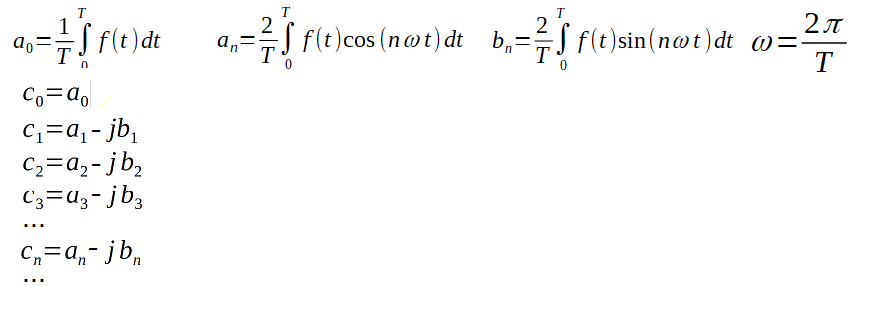Fig. 3-11
Real and complex Fourier coefficients
a0,a1,b1,a2,b2…an, bn… are the same as Fig.2-12 Chapter 2.
When     c0, c1,c2,c3,c4…,cn… coefficients are calculated
then        f(t) real periodic function may be presented as:Fig. 3-12
Complex Fourier Series-General formula with real axis Re z
This is a Fig.3-8a formula  generalisation, where f(t) consists of harmonics only. The number of harmonics may be infinitive in Fig. 3-12! The formula approximates the f(t) function when n=∞ mostly. But finite n, for example n=20 approximates f(t) quite well.
The a0=c0 component is constant. It didn’t exist in Fig.3-8a.
Fig.3-13 shows the f(t) function components as rotating vectors.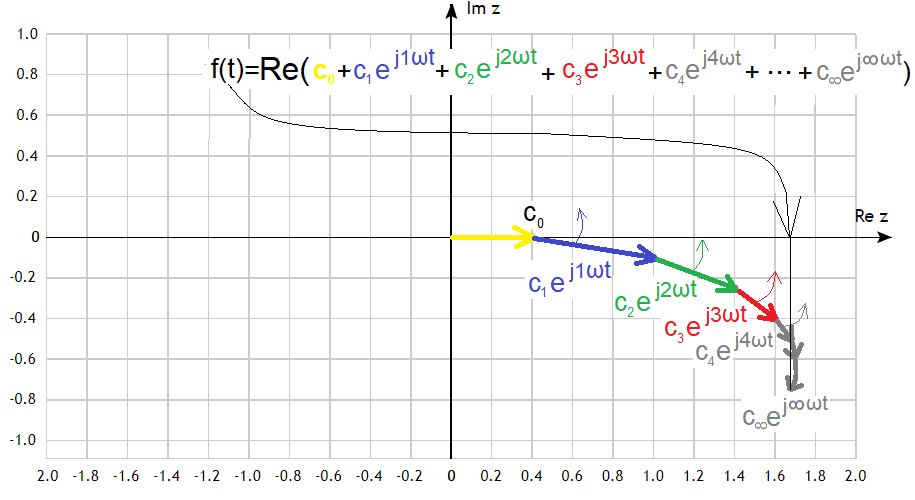Fig.3-13
The yellow vector c0  doesn’t rotate. Or formally rotates with ω=0. The others rotate “each around previous” end. I.e. around c0 constant indirectly. The next vector rotation speed is increasing. The consecutive vectors are smaller and the “final” vector c∞exp(j∞t) aims at mostly. The vertical projection of the “final” vector moves in Re z axis acc. to f(t) function!
Note
The “final” rotating vector may be not c∞exp(j∞t), but for example  c20*exp(j20t) in practice. The f(t) function will be quite well approximated then.

Chapter 3.5.2 Square wave example
The square wave was exactly analyzed as Trigonometric Fourier Series in chapters 2.5 and 2.6. Glance at it for a while.
The square wave with ω=2π (i.e. T=1sec) is approximated as f(t)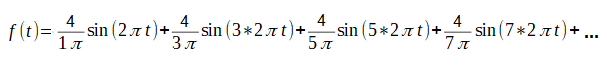Fig. 3-14
Fourier Trigonometric Series
for square wave with ω=2π 1/sec.
The coefficients are calculated acc. Fig.3-11
a0,a1a2a3,a4…=0
b2,b4,b6…=0
b1=4/1π, b3=4/3π, b5=4/5π,b7=4/7π
and the f(t) is as Fig. 3-14
The complex c1, c3, c5, c7 coefficients are calculated then :
c1=a1-jb1=0-4j/π=-4j/π
c3=a3-jb3=0-4j/3π=-4j/3π
c5=a5-jb5=0-4j/5π=-4j/5π
c7=-4j/7π =0-4j/7π=-4j/7π
The Trigonometric Fourier Series of the Square Wave may be presented
as Complex Fourier Series or as rotating vectors when 1ωt=0 and 1ωt=60º.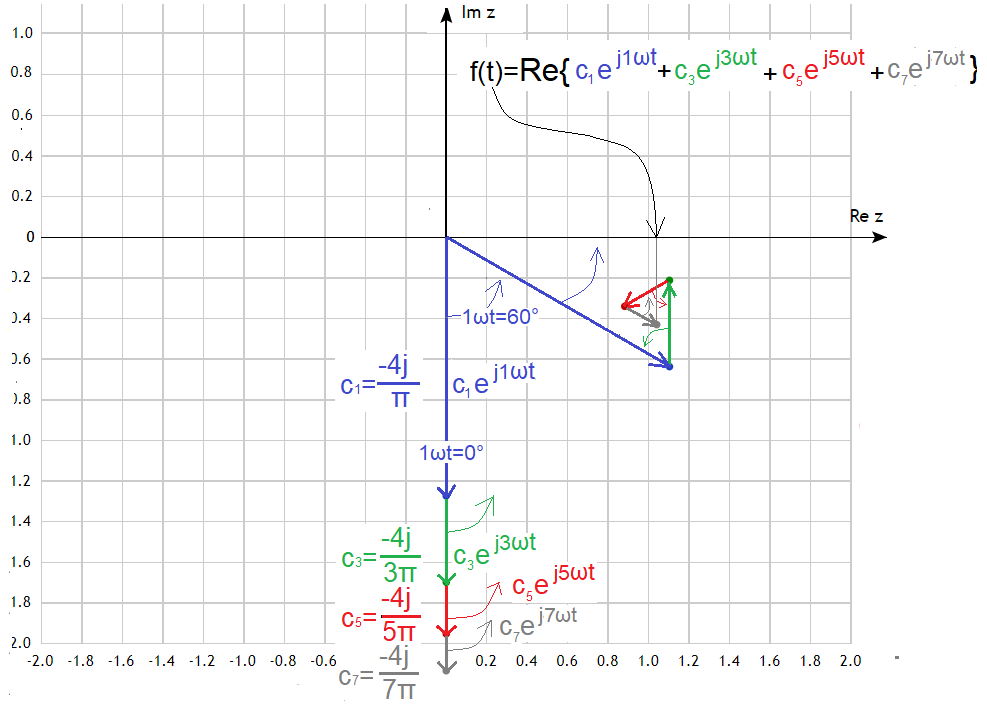Fig. 3-15
Complex Fourier Series
for Square Wave
The c1,c3,c5,c7 i.e. 4 first nonzero coefficients are used only, so the Square Wave  approximation will be not sensational.
You have to imagine the next c9,c11,c13,…c∞ vectors.
There are presented as c1exp(j1ωt),c3exp(j3ωt),c5exp(j5ωt),c7exp(j7ωt) rotating vectors in 2 states:
when 1ωt=0° (initial state)
when 1ωt=60° (or 3ωt=180° or 5ωt=300° or 7ωt=420°)
The fastest is the c7exp(j7ωt) vector and it made the biggest rotation=420°–>full rotation 360°+60°.
The vertical end of the c7exp(j7ωt) vector moves as f(t) square wave   approximation–>see Fig.3-16 YouTube. Why approximation and not ideal square wave? Because it wasn’t “infinte” as c∞exp(j∞ωt) vector. The more vectors are used, the f(t) is more similar to the ideal square wave. Where is the hypothetical c∞exp(j∞ωt) end position? It lays somewhere near c7exp(j7ωt) vector end.

Chapter 3.5.3 Square wave YouTube animation

Fig.3-16
Square wave complex Fourier Series  animation
The higher rotating vectors are the formula components. The c0 constant doesn’t exist here.
f(t)=Re{c1exp(j1ωt)+c3exp(j3ωt)+c5exp(j5ωt)+c7exp(j7ωt)}
The low “Solar System with Sun, Earth, Moon and satellite” is a vectors sum. The additional satellite was included as the fourth  vector. The sinusoids are the rotating vectors equivalents.
The sum of these sinusoids forms square wave appproximation. Trigonometric Fourier Formula with 4 components.
Now stand the computer monitor on the right side (Note for serious people. It’s joke only!) The Re z axis is on the bottom now!
The vertical projection on the of the last satelite of the Solar System is just f(t) square wave approximation presented as
Complex Fourier Series with real axis Re z
f(t)=Re{(-4j/1π)exp(j1ωt)+(-4j/3π)exp(j3ωt)+(-4j/5π)exp(j5ωt)+(-4j/7π)exp(j7ωt)}
The square wave approximation with components isn’t ideal. It’s obviously that for example  20 components are better.

Chapter 3.5.4 Different functions animation
I recommend the wonderful site.  Pierre Guilleminot is an author–>author
You can change the f(t) function and the components number. The bigger is this number, the f(t) approximation is more accurate. Convince Yourself!Fig. 3-17
Pulse simulation example
ANIMATION

Chapter 3.6 Complex Fourier Series “without  real axis Re z” formula
1. f(t) is any perodic function with T period
2.
Calculate  ω=2π/T pulsation
3.
Calculate coefficients
real  a0,a1,b1,a2,b2,…an,bn… and c0 coefficients
complex ..c(-n)…c(-3),c(-2),c(-1),c0,  c1,c2,c3…cn…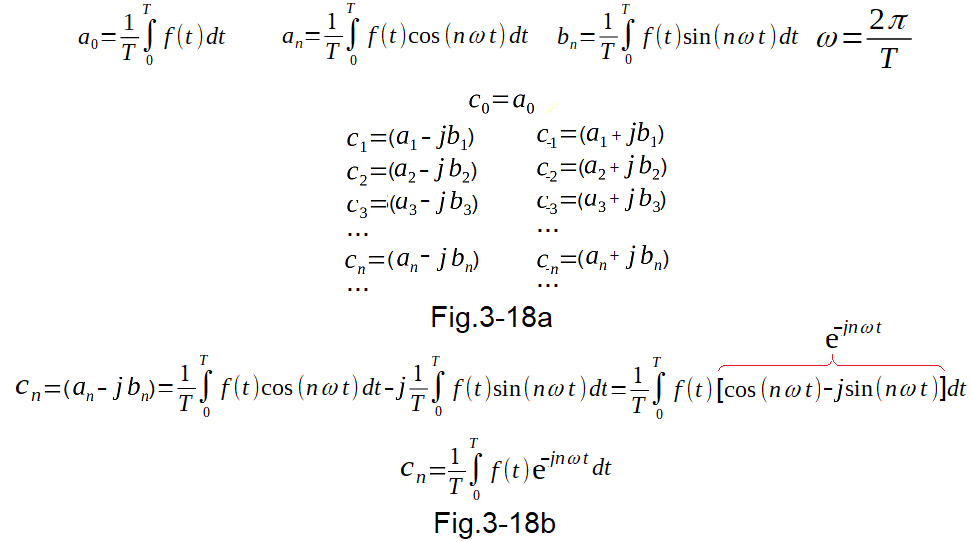Fig. 3-18
Coefficients
You get then
Complex Fourier Series “without  real axis Re z” formula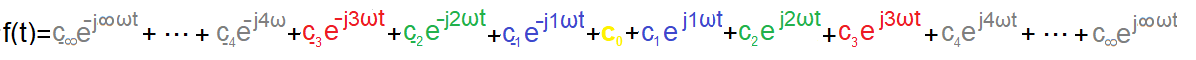Fig. 3-19
Formula
Note
1. a1,b1,a2,b2…an,bn coefficients are halfs of the Fig. 3-11 appropriate a1,b1,a2,b2…an,bn coefficients.
2. There are twice as much complex coefficients as in Fig. 3-11
3. The calculated real f(t) function is absolutely the same as in Fig. 3-11
4. You don’t need to use Re z {…} operand!
The formula may be presented as opposite rotating vectors sum.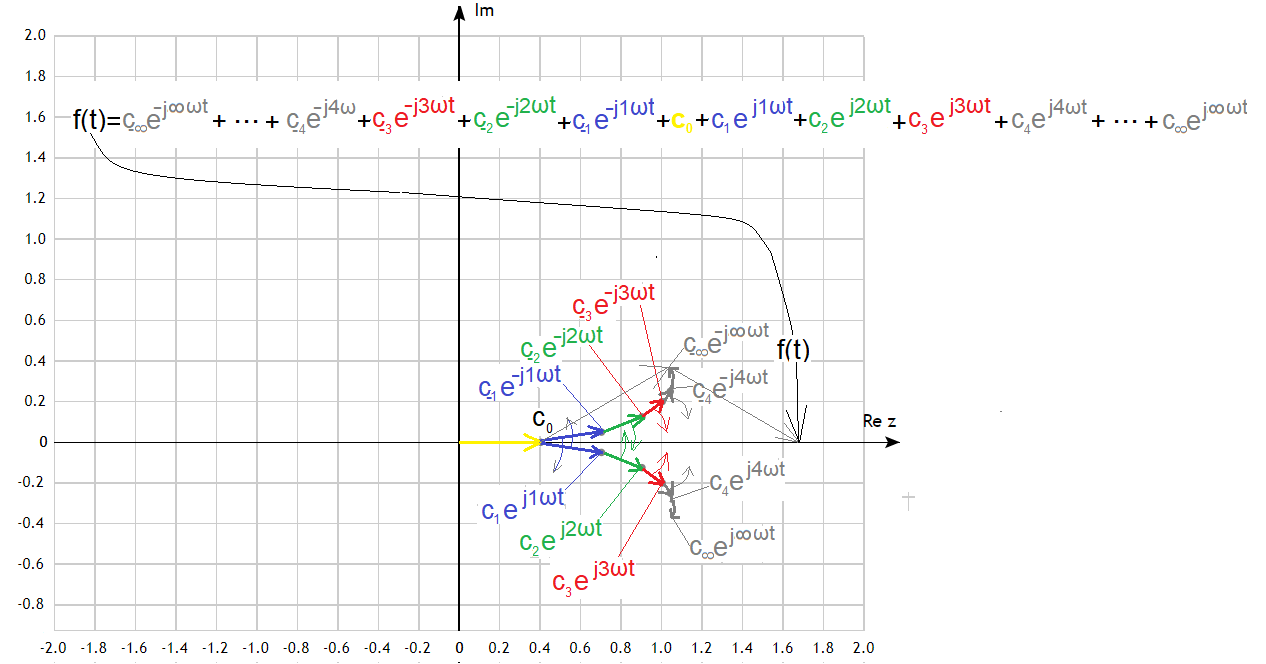Fig. 3-20
Complex Fourier Series as opposite rotating vectors sum.
To be precise “+c0 constant component”
Reason
Every opposite rotating vectors sum of the pair -for example
c1exp(j1ωt)+c(-1)exp(-j1ωt)
is a c1exp(j1ωt) vector of the Fig.3-13 vertical projection for Re z.
Therefore
c1exp(j1ωt)+c(-1)exp(-j1ωt)=a1cos(1ωt)+ b1sin(1ωt)
Therefore
All pairs sum+c0 forms Fig.3-3 and Fig.3-19 formulas
The elegant and condensed form is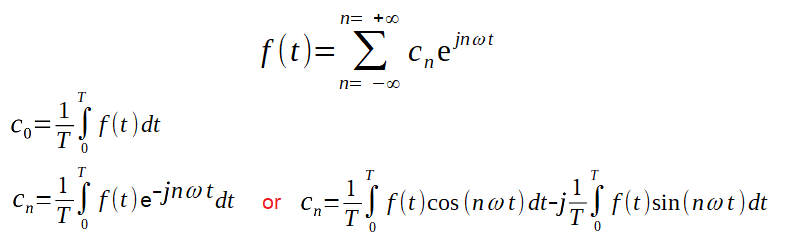Fig. 3-21
Complex Fourier Series
Complex Fourier Series “without  real axis Re z” formula is more popular than “with real axis Re z”.
So it’s simply known a Complex Fourier Series.
By the by
You know that there is positive and negative frequency/pulsation.
Rotating anticlocwise vectors have positive frequency, clocwise vectors have negative frequency.
This intrigued me once. I could imagine that direct current frequency f=0.
But negative frequency? Is something more constant than constant? I know the answer now!